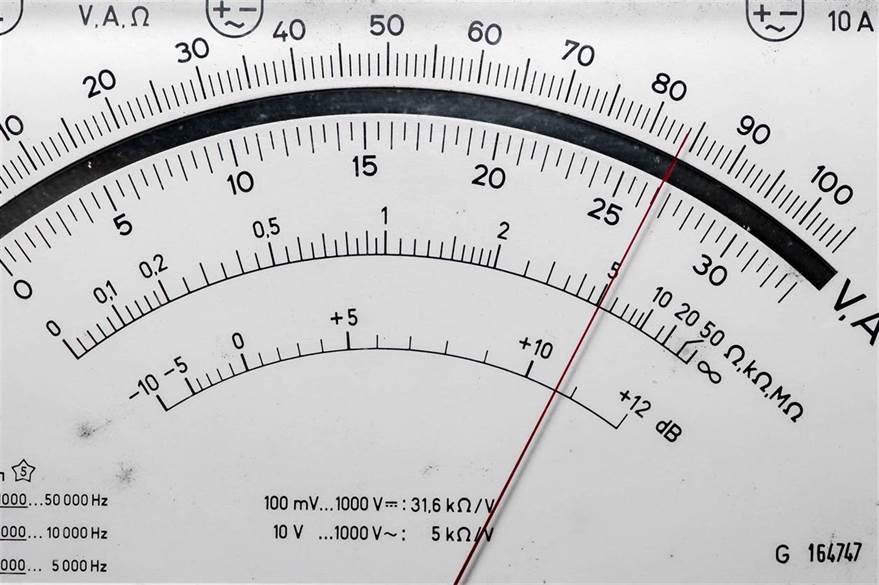Select PageElectricity is omnipresent, but the average citizen doesn’t necessarily understand exactly how it works. That’s not surprising at all; the electron was only discovered in 1897, just over 100 years ago. No one expects everyone to understand precisely how electricity works; it’s extraordinarily complicated, and to begin to fully grasp the concept takes years of schooling. That said, having a basic understanding of how electricity is measured will help you have a better grasp of how it works.

The best analogy for electricity is water; while the two are quite different, the way they flow can be used to illustrate a few key concepts. The first is voltage, which is the difference in charge between two points on an electrical circuit. The water analogy for this is pressure; imagine a water tank with a lot of water in it pouring into a pipe with no water at the end. There’s a difference in pressure between the two points; decrease the amount of water in the tank, the pressure goes down. Decrease the charge on one of the points of an electrical circuit, the voltage goes down.

The amount of water flowing through the pipe is analogous to an electrical current. Current is measured in Amperes, usually just called Amps. The more Amps of current, the more charge is flowing through the circuit at any given time. That means if the voltage is increased, the current is increased as well; more pressure means more water flowing through the pipes, as it were.

That leads us to the third concept that we’re going to touch on for today, which is resistance. This particular concept translates quite well between water and electricity. Imagine your water pipe becomes half as wide; now, only half as much water can pass through it at any given time, even if the pressure remains the same. That means the flow of water has been reduced even though the pressure is constant; in the same way, if you have the same amount of voltage, but there’s twice as much resistance in your circuit, less charge will flow between the two points.

So, we have three key concepts to go over:

Pressure=Voltage (V) (Measured in Volts, V)

Flow=Current (I) (Measured in Amps, A)

Resistance=Resistance (R) (Measured in Ohms, Ω)

Using the concepts we’ve learned so far, we can establish that an increase in voltage increases the current, and that an increase in resistance decreases the current. That might gives us an equation that looks like: Current = Voltage/Resistance, or I = V/R. That’s one of the most important concepts in electricity, Ohm’s Law. Thanks for the analogy, water!

You don’t have to know all of this, but your team of qualified electricians had better know this and more! Electricity is incredibly complicated, but it’s ubiquity means that a basic understanding is important, if for no other reason than to sound smart in front of your friends.